Advanced Database Management System - Tutorials and Notes: How to calculate transition and emission probabilities in HMM

Question:

## Given the following tagged corpus as the training corpus, answer the following questions using Maximum Likelihood Estimation (MLE);

Training corpus:
But/CC then/RB the/DT bear/NN thought/VBD that/IN the/DT fish/NN was/VBD too/RB small/JJ to/TO fill/VB the/DT stomach/NN of/IN bear/NN. He/PRP decided/VBD to/TO catch/VB a/DT bigger/JJR fish/NN. He/PRP let/VBD off/RP the/DT small/JJ fish/NN and/CC waited/VBD for/IN some/DT time/NN. Again/RB a/DT small/JJ fish/NN came/VBD and/CC he/PRP let/VBP it/PRP go/VB thinking/VBG that/IN the/DT small/JJ fish/NN would/MD not/RB fill/VB his/PRP\$ belly/NN. This/DT way/NN he/PRP caught/VBD many/JJ small/JJ fish/NN, but/CC let/VB all/DT of/IN them/PRP go/VB off/RP. By/IN sunset/NN, the/DT bear/NN had/VBD not/RP caught/VBN any/DT big/JJ fish/NN.
 Tags used in this corpus CC – Conjunction DT – Determiner IN - Preposition JJ – Adjective JJR – Adjective comparative MD - Modal NN – Noun PRP – Personal pronoun PRP\$ - Possessive pronoun RB – Adverb RP - Particle TO - To VB - Verb VBD – Verb past tense VBN – Verb past participle VBP – Verb non-3rd person singular present

(a) Find the tag transition probabilities using MLE for the following.
(i) P(JJ|DT)                   (ii) P(VB|TO)               (iii) P(NN|DT, JJ)
(b) Find the emission probabilities for the following;
(i) P(go|VB)        (ii) P(fish|NN)

### (a) We can compute the maximum likelihood estimate of bigram and trigram transition probabilities as follows;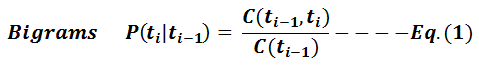In Equation (1),
• P(ti|ti-1) – Probability of a tag ti given the previous tag ti-1.
• C(ti-1, ti) – Count of the tag sequence “ti-1 ti” in the corpus. That is, how many times tag ti follows the tag ti-1 in the corpus.
• C(ti-1) – Count of occurrence of tag ti-1 in the corpus. That is, frequency of the tag ti-1 in the corpus.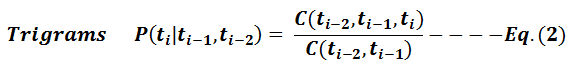In Equation (2),
• P(ti|ti-1, ti-2) – Probability of a tag ti given the previous two tag ti-1, and ti-2.
• C(ti-2, ti-1, ti) – Count of the tag sequence “ti-2 ti-1 ti” in the corpus. That is, how many times tag ti follows the couple of tags ti-2 and ti-1 in the corpus.
• C(ti-2, ti-1) – Count of occurrence of tag sequence “ti-2 ti-1” in the corpus.

Solution to exercise a(i):

### Find the probability of tag JJ given the previous tag DT using MLE

To find P(JJ | DT), we can apply Equation (1) to find the bigram probability using MLE.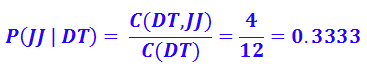In the corpus, the tag DT occurs 12 times out of which 4 times it is followed by the tag JJ.

Solution to exercise a(ii):

### Find the probability of tag VB given the previous tag TO using MLE

To find P(VB | TO). We can apply Equation (1) to find the bigram probability using MLE.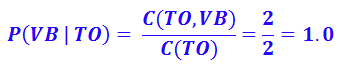In the corpus, the tag TO occurs 2 times out of which 2 times it is followed by the tag VB.

Solution to exercise a(iii):

### Find the probability of tag NN given previous two tags DT and JJ using MLE

To find P(NN | DT JJ), we can apply Equation (2) to find the trigram probability using MLE.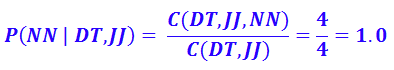In the corpus, the tag sequence “DT JJ” occurs 4 times out of which 4 times it is followed by the tag NN.

### (B) We can compute the Maximum Likelihood Estimate of emission probability as follows;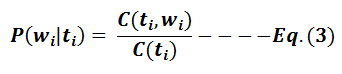In Equation (3),
• P(wi|ti) – Probability of a word wi given the tag ti which is associated with the word.
• C(ti, wi) – Count of occurrence of word wi with associated tag ti in the corpus. C(ti) – Count of occurrence of tag ti in the corpus.

Solution to exercise b(i):

### Find the Maximum Likelihood Estimate of emission probability P(go|VB)

To find the MLE of emission probability P(go | VB), we can apply Equation (3) as follows;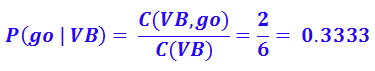In the corpus, the tag VB occurs 6 times out of which VB associated with the word “go” 2 times. [How to read P(go | VB)? – If we are going to generate a tag VB, how likely it will be associated with the word go]

Solution to exercise b(ii):

### Find the Maximum Likelihood Estimate of emission probability P(fish|NN)

To find the MLE of emission probability P(fish | NN), we can apply Equation (3) as follows;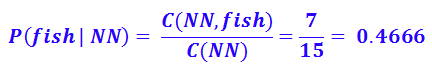In the corpus, the tag VB occurs 6 times out of which VB associated with the word “go” 2 times. [How to read P(go | VB)? – If we are going to generate a tag VB, how likely it will be associated with the word go]

**********